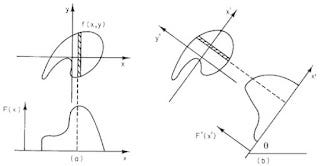# The Central Slice Theorem: An ExampleFig. 12.12 from IPMB, showing how to do a projection.

## The Projection

Figure 12.12 shows that the projection is an integral of the object along various lines in the direction θ, as a function of displacement perpendicular to each line, x’. The integral becomes

## The One-Dimensional Fourier Transform

The next step is to evaluate the one-dimensional Fourier transform of the projection

## Two-Dimensional Fourier Transform

To calculate the two-dimensional Fourier transform, we must evaluate the double integral

## Select One Direction in Frequency Space

If we want to focus on one direction in frequency space, we must convert to polar coordinates: kx = k cos θ and ky = k sin θ. The result is

--

--